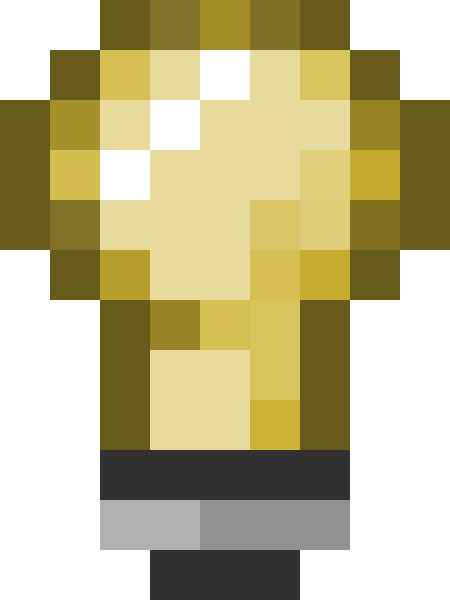# User:TomEdwards/Vector draft

Vector is a C++ class that represents a line with direction and length, starting at the current origin.

Each vector contains three `vec_t` 'ordinates' (which are just redefined floats). `(0,20,5)` is a Vector that ends 20 units north of and 5 units above the current origin.Note:Source's vector class is a vector in the geometric sense of the word, and is very different to the Standard Template Library's, which is a type of array. The STL-style vector has been renamed `CUtlVector` in Source.

## Declaration

```Vector vecMyVector = Vector(0,20,5);
```

You could also assign to the X, Y and Z member variables separately.

## Uses

### Positioning

Every entity's position is stored as a vector relative to its parent. You are likely to be familiar with this idea already as grid coordinates.

The parent-relative vector stored by the entity is 'local'; calculating an 'absolute' vector relative to the world requires extra work. The two Abs functions below do that work for you, but are more expensive.

• `GetAbsOrigin()`, `SetAbsOrigin()`
• `GetLocalOrigin()`, `SetLocalOrigin()`

### Movement

An entity attempts to move the length of its velocity vector once per second. The code looks something like this:

```Vector velocity = Vector(0,5,0); // 5 units/second forward

Vector vecNewOrigin = GetAbsOrigin() + velocity * gpGlobals->frametime; // frametime is in seconds, e.g. 0.033

SetAbsOrigin(vecNewOrigin);
```

Notice how `frametime` is used to regulate the entity's speed regardless of how long each frame takes to calculate. See scalar multiplication for more detail on the operation.

As with the origin, velocity is stored relative to the entity. To do: What does AbsVelocity mean?

• `GetAbsVelocity()`, `SetAbsVelocity()`
• `GetLocalVelocity()`, `SetLocalVelocity()`

### Tracelines

Main article: TraceLines

Tracing is the process of going from a point A towards another point B, and finding out the first thing that we "hit" on our way from A to B (TraceLine).

## Operations

All vectors in an operation must have the same origin for the result to make sense. Whether a local or absolute origin is used depends on what you're trying to achieve.

Adding two (or more) vectors combines them. You have already experienced vector addition if you've ever pushed an object with two hands!

### Subtraction

Subtracting one vector from another produces the difference between the two - in other words, how to get to the first location from the second. The result is local to the second vector.Tip:The order in which you subtract defines the direction of the vector.

### Multiplication

#### Scalar

Multiplying or dividing a vector by a scalar (i.e. an int or float) will change its length (sometimes called "magnitude") without affecting its direction.Tip:Dividing a vector by its length normalises it. Use `VectorNormalize()` to do this quickly.

#### Dot product

Multiplying two vectors then adding the result's ordinates produces a dot product, which when both vectors have been normalised (see above) is equal to the cosine of the angle between the two vectors. One use of a dot product is to tell how closely the direction of two vectors match. +1 means a match, 0 means they are perpendicular to each other, and -1 means they are opposed.Note:True dot products are only produced when the length of both vectors is 1. The normalisation step has been skipped in the following demonstration to make its equations simpler (but the positive/zero/negative rule still applies).

This code calculates a dot product with the aid of Source's various helper functions:

```Vector vecTarget = GetAbsOrigin() - pTarget->GetAbsOrigin();	// Get local vector to target
VectorNormalize(vecTarget);	// Normalisation needs to be done beforehand

Vector vecFacing;
AngleVectors(GetLocalAngles(),&vecFacing);	// Convert facing angle to equivalent vector (arrives normalised)

float result = DotProduct(vecTarget,vecFacing);	// Get the dot product.

if (result > 0)
Msg("pTarget is in front of me!\n");
```Tip:There is no need to normalise if you only care about whether one location is in front of another.

#### Cross product

A cross product is a vector perpendicular to two input vectors. The cross product of a vector pointing down the X-axis and a vector pointing down the Y-axis is a vector pointing down the Z-axis. It's used to extrapolate a third dimension from just two.

The equation is fiddly and doesn't have to be learnt; just use `CrossProduct()`. There generally isn't any need to normalise the input vectors.

## Member functions

`float/vec_t Length()`
`vec_t VectorLength(const Vector&)`
Returns the vector's length, in units.
`vec_t LengthSqr()`
Returns length squared.
`vec_t Length2D()`
Returns the length, disregarding the up-element.
`vec_t Length2DSqr()`
Returns the length squared, disregarding the up-element.
`void Negate()`
Reverses the vector's direction without affecting its length.
`vec_t DotProduct(const Vector& a, const Vector& b)`
To do: Someting to do with determining how closely two vectors match.
`void CrossProduct(const Vector& a, const Vector& b, Vector& result )`
To do: Something to do with creating a surface normal.
`bool WithinAABox( Vector const &boxmin, Vector const &boxmax)`
Does the Vector intersect this axis-aligned box? To do: Are argument vectors local or abs?

## Helper functions

• `UTIL_VecToYaw()`, `UTIL_VecToPitch()`
• `AngleVectors()`
• `VectorAngles()`
• `VectorNormalize()`